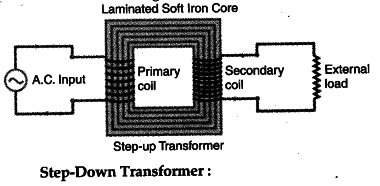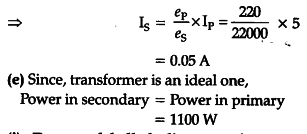# Write the function of a transformer

(i) Write the function of a transformer. State its principle of working with the help of a diagram, Mention various energy losses in this device. (ii) The primary coil of an ideal step up transformer has 100 turns and transformation ratio is also 100. The input voltage and power are respectively 220 V and 1100 W Calculate
(a) number of turns in secondary
(b) current in primary
(d) current in secondary
(e) power in secondary

(i) Function : Transformer is used for converting
low alternating voltage at high current into high voltage at low current and vice-versa.
Principle : It works on the principle of mutual induction.
Various Energy loses in transformer are -
(a) Flux losses.
(b) Copper and Iron losses.